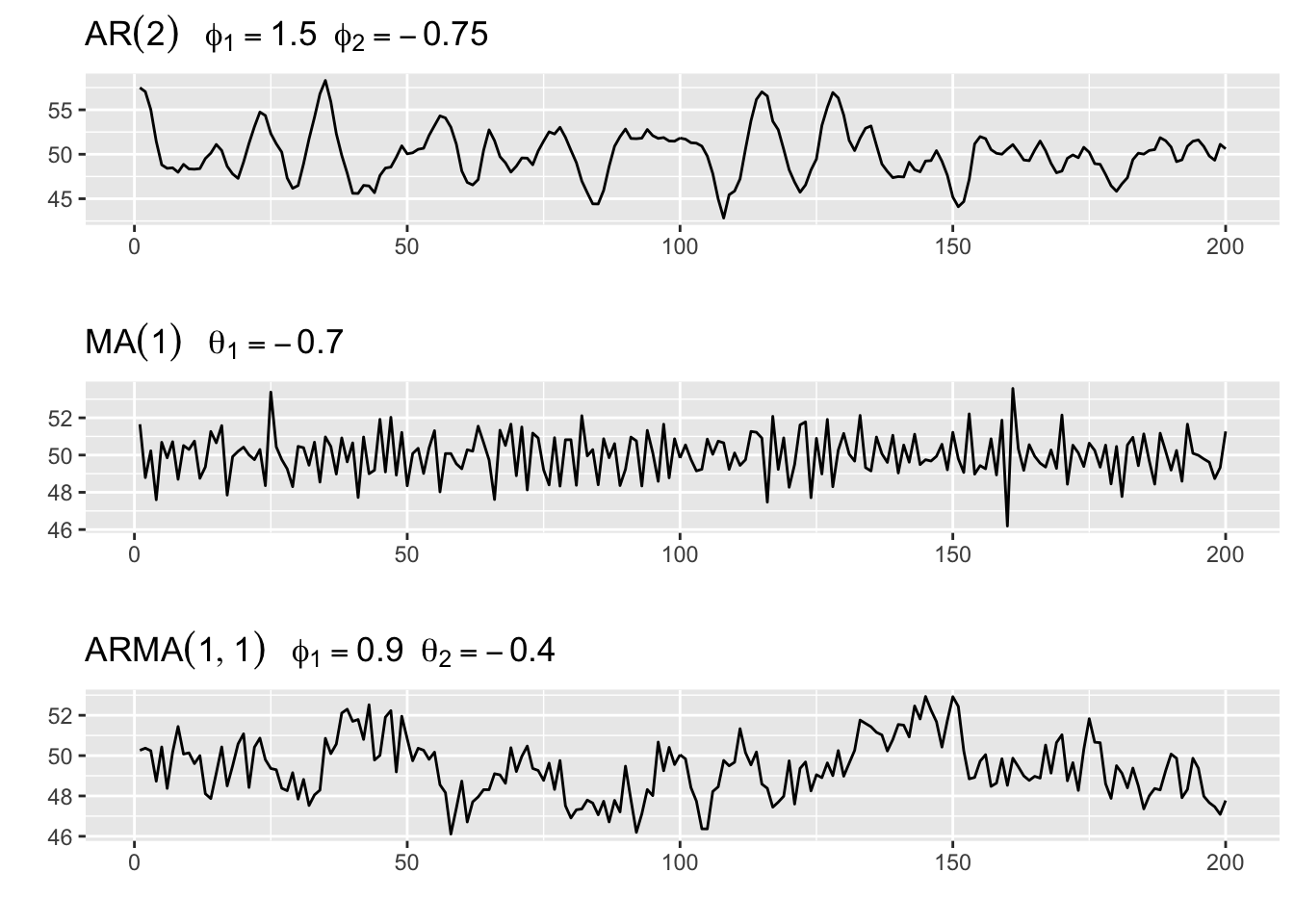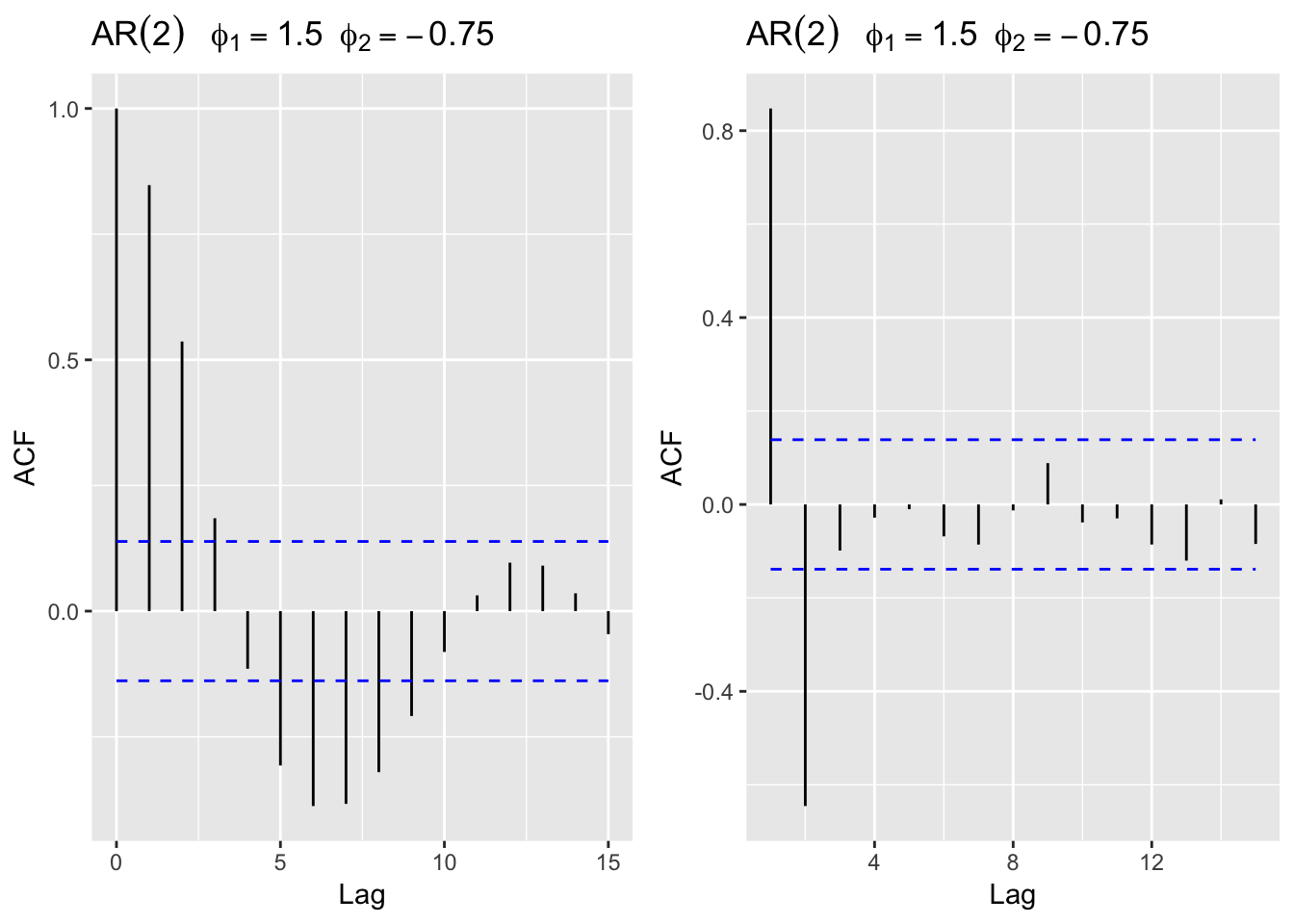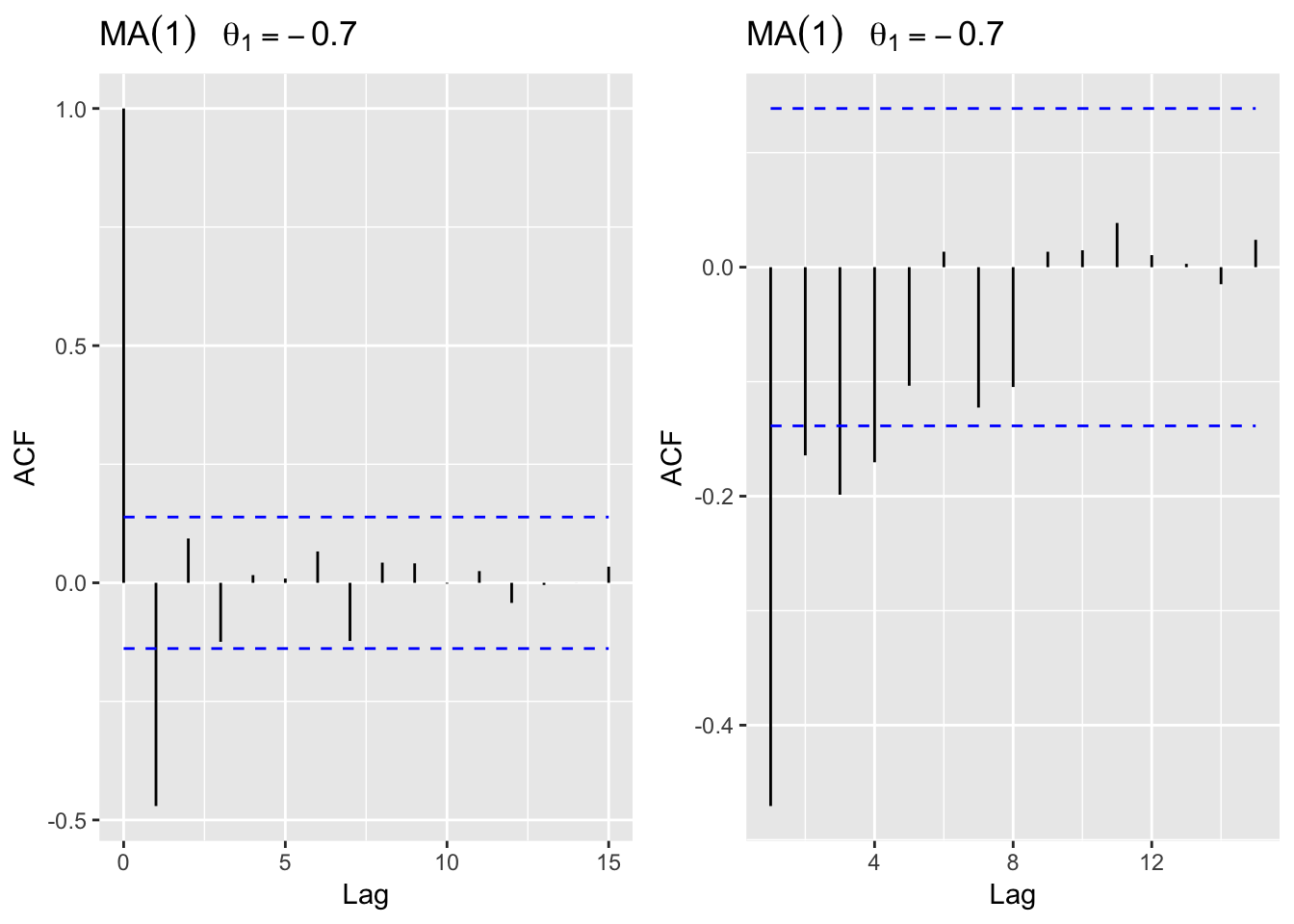We can combine the autoregressive (AR($$p$$)) and moving average (MA($$q$$)) processes to form a mixed autoregressive moving average process as follows

$y_t = \underbrace{\phi_1y_{t-1}+...+\phi_p y_{t-p}}_{\text{AR}} + \underbrace{\theta_iw_{t-1}+...+\theta_qw_{t-q}}_{\text{MA}}\text{,}$ with $$\phi_p \ne 0$$, $$\theta_q \ne 0$$, and $$\sigma^2_w >0$$. The equation can be rewritten as

$y_t = \underbrace{\sum_{j=1}^p\phi_jy_{t-j}+ w_t}_{\text{AR}} + \underbrace{\sum_{j=0}^q\theta_jw_{t-j}}_{\text{MA}}\text{,}$

The term is called an autoregressive-moving average series of order ($$p$$, $$q$$), or an ARMA($$p$$, $$q$$) series. When $$q = 0$$, the model is called an autoregressive model of order $$p$$, AR($$p$$), and when $$p = 0$$, the model is called a moving average model of order $$q$$, MA($$q$$). A slightly more general definition of an ARMA process incorporates a nonzero mean value $$\mu$$, and can be obtained by replacing $$y_t$$ by $$y_t-\mu$$ and $$y_{t-j}$$ by $$y_{t-j}-\mu$$ in the equation above.

ARMA model may be seen as a regression of the present outcome $$(y_t)$$ on the past outcomes $$(x_{t-1}, ..., y_{t-p})$$ with correlated errors.

#### The Partial Autocorrelation Function

The autocorrelation function of an MA series cuts of sharply whereas those for AR and ARMA series exhibit exponential decay (with possible sinusoidal behavior superimposed). This makes it difficult to distinguish between an ARMA series and a purely AR series by plotting only its autocorrelation function. Hence, the partial autocorrelation function (PACF) is used as it behaves like the ACF of MA models, but for AR models.

The partial autocorrelation function (PACF) of a stationary process, $$y_t$$, denoted $$\phi_{kk}$$, for $$k=1,2,...,$$ is

$\phi_{kk} = \text{cor}(y_k-\hat y_k, y_0-\hat y_0), \quad k \ge 2\text{,}$ where $$\hat y_k$$ is the regression of $$y_k$$ on $$\{y_1, y_2,...,y_{k-1}\}$$. The PACF, $$\phi_{kk}$$, is the correlation between $$y_{t+k}$$ and $$y_t$$ with the linear dependence of every one between them, namely $$\{y_{t+1},y_{t+2},...,y_{t+k-1} \}$$, removed. In other words the partial autocorrelation is the correlation between $$y_s$$ and $$y_t$$ with the linear effect of everything in the middle removed. The computation of PACF is done recursively via the Durbin-Levinson algorithm.

The PACF for MA models behaves much like the ACF for AR models. Also, the PACF for AR models behaves much like the ACF for MA models. The behavior of ACF and PACF for ARMA models is summarized (Shumway and Stoffer 2011 and Wei 2006).

$\begin{array}{l|lll} & \text{AR}(p) & \text{MA}(q) & \text{ARMA}(p,q) \\ \hline \text{ACF} & \text{Tails off}^\text{1} & \text{Cuts off after lag }q & \text{Tails off after lag (}q-p\text{)} \\ \text{PACF} & \text{Cuts off after lag }p & \text{Tails off}^\text{1} & \text{Tails off after lag (}p-q\text{)}\\ \hline \end{array}$ $$^\text{1}$$Tails off as exponential decay or damped sine wave.

If the ACF exhibits slow decay and the PACF cuts off sharply after lag $$p$$, we would identify the series as AR$$(p)$$. If the PACF shows slow decay and the ACF show a sharp cutoff after lag $$q$$, we would identify the series as being MA($$q$$). If both the ACF and PACF show slow decay we would identify the series as being mixed ARMA.

#### Simulation of an ARMA process

In this section we simulate AR, MA and ARMA processes and review their autocorrelational structures. Therefore we apply the arima.sim() function and build three different models, each with a mean of 50:

AR(2) model: $$y_t = 50 + 1.5(y_{t-1}-50)-0.75(y_{t-2}-50)+ w_t$$

MA(1) model: $$y_t = 50 + w_t-0.7w_{t-1}$$

ARMA(1,1) model: $$y_t = 50+ 0.9(y_{t-1}-50)+ w_t-0.4w_{t-1}$$

set.seed(250)
arma.20 <- arima.sim(list(order = c(2,0,0),
ar = c(1.5, -0.75)),
n = 200) + 50
arma.01 <- arima.sim(list(order = c(0,0,1),
ma = -0.7),
n = 200) + 50
arma.11 <- arima.sim(list(order = c(1,0, 1),
ar = 0.9,
ma = -0.4),
n = 200) + 50

In the next step we plot each model for 200 time steps.

library(ggfortify)
library(gridExtra)

p1 <- autoplot(arma.20)+
ggtitle(expression(AR(2)~~~phi==1.5 * ~~phi==-0.75))
p2 <- autoplot(arma.01)+
ggtitle(expression(MA(1)~~~theta==-0.7))
p3 <- autoplot(arma.11)+
ggtitle(expression(ARMA(1,1)~~~phi==0.9 * ~~theta==-0.4))
grid.arrange(p1, p2, p3, ncol = 1)Now we review for each of the three models the correlational structure.

AR(2) process

Based on the table from above we expect the ACF to tail off and the PACF to cut off after lag $$q=2$$. We use the acf() function to calculate the autocorrelation function and the pacf() function to calculate the partial autocorrelation function.

## ACF
p1 <- autoplot(acf(arma.20,
plot = F,
lag.max = 15)) +
ggtitle(expression(AR(2)~~~phi==1.5 * ~~phi==-0.75))

## PACF
p2 <- autoplot(acf(arma.20,
plot = F,
lag.max = 15,
type = 'partial')) +
ggtitle(expression(AR(2)~~~phi==1.5 * ~~phi==-0.75))
grid.arrange(p1, p2, ncol = 2)MA(1) process

Based on the table from above we expect the ACF to cut off after lag $$q=1$$ and the PACF to tail off.

## ACF
p1 <- autoplot(acf(arma.01,
plot = F,
lag.max = 15)) +
ggtitle(expression(MA(1)~~~theta==-0.7))

## PACF
p2 <- autoplot(acf(arma.01,
plot = F,
lag.max = 15,
type = 'partial')) +
ggtitle(expression(MA(1)~~~theta==-0.7))
grid.arrange(p1, p2, ncol = 2)ARMA(1,1) process

Based on the table from above we expect the ACF and the PACF to tail off.

## ACF
p1 <- autoplot(acf(arma.11,
plot = F,
lag.max = 15)) +
ggtitle(expression(ARMA(1,1)~~~phi==0.9 * ~~theta==-0.4))

## PACF
p2 <- autoplot(acf(arma.11,
plot = F,
lag.max = 15,
type = 'partial')) +
ggtitle(expression(ARMA(1,1)~~~phi==0.9 * ~~theta==-0.4))
grid.arrange(p1, p2, ncol = 2)